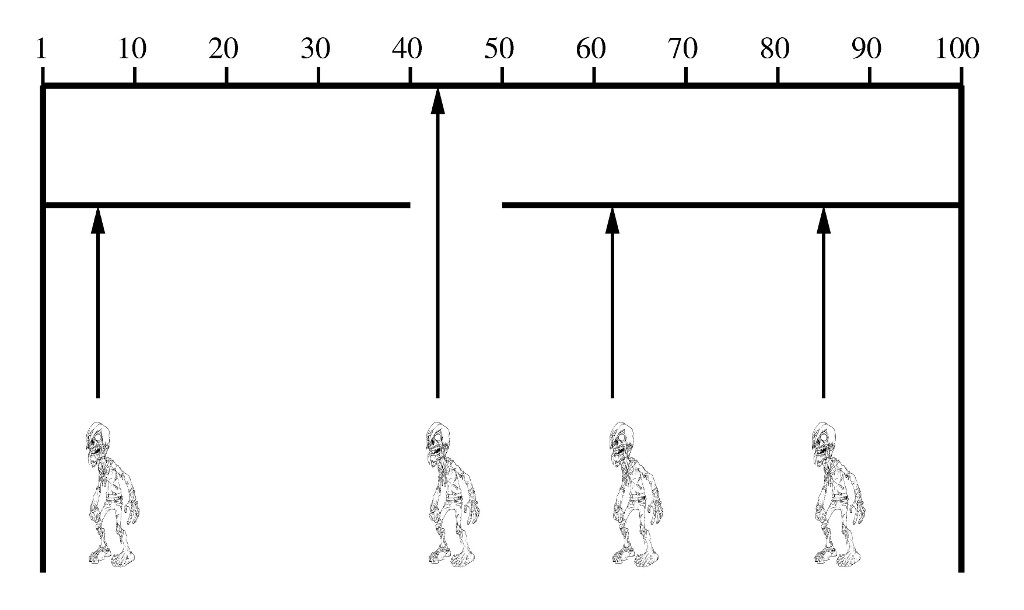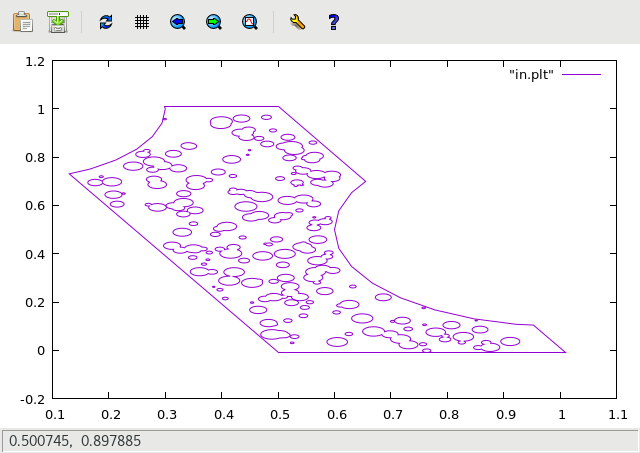Run!!!

Zombies are pretty cool.  This post describes something a little less cool, but uses zombies to explain the concept (in a shallow, transparent attempt to capture your attention!)

# Zombie Door Method

Imagine we want to generate a uniformly distributed random sampling in some complex space that our random number generator does not directly support.Let me start with an simple example.  Imagine we have a random number generator that produces a random integer between 1 and 100.  However, we actually want to generate random numbers between 41 and 50.  (I know there are better ways to do this, but stick with me for this example.)  Let’s solve this with the zombie door method.

• Build a wall and label it 1-100 where 1 is to the far left, and 100 is to the far right.
• Cut a door in your wall from 41 to 50.
• Now create random zombies starting anywhere from 1 to 100 and let them walk straight towards the wall.
• The zombies will lurch across the room and eventually hit wall, explode, and dissolve in a fizzing puddle of bloody goo … or whatever it is that zombies do when they die.
• The zombies that are lucky enough to walk through the door survive!

For this example it would be easy to scale and offset the usually available random number generator that produces a floating point value between 0 and 1, but it illustrates the basic approach of the ‘zombie door’ method.

# A More Interesting Example

Imagine we have an arbitrary polygon outline and we want to splatter it with random circles.  However, we only want circles that are completely within the polygon.  (And we want our random circles to be a true  unbiased, uniformly distributed random sample.)  This example is just like the simple ‘wall’ example except now we have gone ‘2D’.

Imagine an arbitrary polygon shape:We would like to fill this shape with 200 random circles, making sure none of our circles straddle the boundary or lie outside the polygon.  We want an even distribution over the interior area of our polygon.

We can do this by generating random circles within the min/max range of our shape, and then testing if they lie completely inside the polygon.  We reject any outliers and keep the inliers.  It’s very simple, but very cool because … you know … zombies!  Here is a picture of how our circles turned out:If you are at all curious as to what became of our dead zombies (all the ones that splatted against the wall) here is the picture of that:If you the reader are concerned that I am making light of a very real pending zombie apocalypse issue then here is my best tip for protecting your residence:Finally, here is the python script I used to generate the pictures in this posting.  I leverage the very, very, very cool GPC polygon clipping library to test if the outline polygon ‘covers’ the circle.

```#!/usr/bin/python

import random

# polygon (GPC) python package: https://pypi.python.org/pypi/Polygon2
import Polygon
import Polygon.IO
import Polygon.Shapes

# this will seed the random number generator with current time and
# force different results on each run.
random.seed()

# this is the bounds of the shape that will contain our splatters
outline = [ [0.5, -0.01], [1.01, -0.01], [0.5, 1.01], [-0.01, 1.01] ]

# define circle properties
num_circles = 200
min_r = 0.002
max_r = 0.02

# no closer than this to the boundary
margin = 0
#margin = 0.002

# create the polygon template (outline)
template = Polygon.Polygon(outline)

# make the shape a bit more interesting
c = Polygon.Shapes.Circle(radius=0.4, center=(1, 0.5), points=32)
template = template - c
c = Polygon.Shapes.Circle(radius=0.3, center=(0, 1), points=32)
template = template - c

# determine max/min of template
min_x = max_x = outline
min_y = max_y = outline
for p in outline:
if p < min_x: min_x = p
if p > max_x: max_x = p
if p < min_y: min_y = p
if p > max_y: max_y = p

print 'template bounds:', min_x, min_y, 'to', max_x, max_y
print 'margin:', margin
print 'num circles:', num_circles

# generate splats using zombie door method
circles = []
while len(circles) < num_circles:
x = random.uniform(min_x, max_x)
y = random.uniform(min_y, max_y)
r = random.uniform(min_r, max_r)

# make the circle
c = Polygon.Shapes.Circle(radius=r, center=(x, y), points=32)

# make the circle padded with extra margin
cm = Polygon.Shapes.Circle(radius=(r+margin), center=(x, y), points=32)

if template.covers(cm):
# circle + margin fully contained inside the template
circles.append(c)
else:

# assemble final polygons and write output
final = Polygon.Polygon()
for c in circles:
final += c
Polygon.IO.writeGnuplot('in.plt', [template, final])
Polygon.IO.writeSVG('in.svg', [final], fill_color=(0,0,0))

reject = Polygon.Polygon()